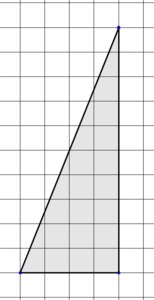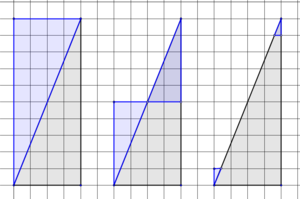# Areas of Right Triangles

Alignments to Content Standards: 6.G.A.1

1. Explain why the right triangle shown has an area of exactly 20 square units.2. The "legs" of a right triangle are the two sides that form the right angle. If one leg of a right triangle is 5 units long, explain what else would have to be true about the right triangle in order for its area to be 30 square units.
3. Here are leg measurements for more right triangles. What is the area of each?
1. 6 and 3
2. 12 and $4 {1\over2}$
3. 3 and 7
4. 6.5 and 9
4. Explain in words how you can find the area of a right triangle when you know the lengths of its legs.
5. Let a represent the length of one leg of a right triangle and b represent the length of the other leg of the right triangle. Write a mathematical expression for the area of the right triangle in terms of a and b.

## IM Commentary

This task is intended to help build understanding as students work toward deriving a general formula for the area of any triangle. The purpose of this task is for students to use what they know about area and express regularity in repeated reasoning (MP.8) to generate a formula for area of a right triangle.

This task is intended to be used before students know that the area of a triangle can be expressed with bh/2. So, in part (c), they should be reasoning using geometric methods (surround and subtract, composing and decomposing, counting unit squares, etc.). They are not expected to use a formula in part (c), because they are not expected to know a formula yet.

It would be appropriate to make grid paper, straight edges, and even scissors available to students who want to think about area in terms of counting square units. In part (c), this tactic will become more difficult, nudging students to think more abstractly.

Students thinking very concretely about the number of unit squares may struggle with parts of (c). For these students, it may be helpful to assist drawing pictures of all four part (c) triangles with leg lengths labeled. Even though it is difficult to count unit squares, students can still reason that the right triangle is half of an associated rectangle, and compute half the area of the rectangle. Generalizing this process will help them respond to parts (d) and (e).

Still other students may simply need support multiplying fractions.

## Solution

1. Answers may vary. Techniques include "completing" the rectangle with sides of length 4 and 10 and arguing that half of its area must be 20 square units, chopping off and relocating a chunk of the right triangle to create a rectangle whose area is 20 square units, and "completing" individual unit squares to create a total of 20 unit squares. Here are some illustrations associated with these methods:2. The other leg would have to measure 12 units in length. A 12 by 5 rectangle would have an area of 60 square units. Slicing this rectangle along a diagonal would create right triangles with legs of 12 and 5 with areas of 30 square units.
3.
1. 9
2. 27
3. $21\over2$or $10{1\over2}$or 10.5
4. 29.25 or 117/4
4. Answers may vary. Examples: Multiply the lengths of the legs together, and then divide by 2. One half of the product of the lengths of the legs.
5. $ab\over2$, ${1\over2}ab$ , $a\times b \div 2$ , or equivalent